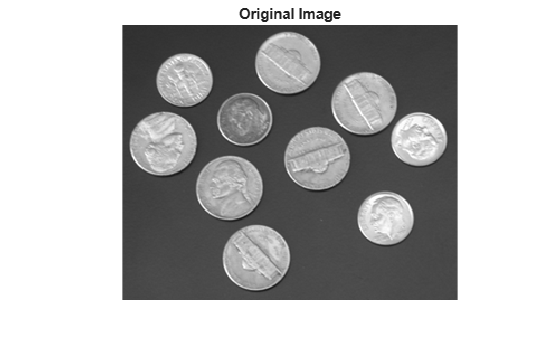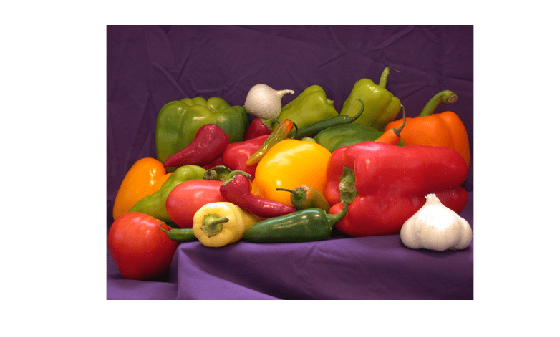# Filter Grayscale and Truecolor (RGB) Images Using imfilter Function

This example shows how to filter a 2-D grayscale image with a 5-by-5 filter containing equal weights (often called an averaging filter) using `imfilter`. The example also shows how to filter an truecolor (RGB) image with the same filter. A truecolor image is a 3-D array of size m-by-n-by-3, where the last dimension represents the three color channels. Filtering a truecolor image with a 2-D filter is equivalent to filtering each plane of the image individually with the same 2-D filter.

There are several MATLAB® functions that perform 2-D and multidimensional filtering that can be compared to `imfilter`. The function `filter2` performs two-dimensional correlation, `conv2` performs two-dimensional convolution, and `convn` performs multidimensional convolution. However, each of these filtering functions always converts the input to `double`, and the output is always `double`. Also, these MATLAB® filtering functions always assume the input is zero padded, and they do not support other padding options. In contrast, `imfilter` does not convert input images to `double`. The `imfilter` function also offers a flexible set of boundary padding options.

### Filter 2-D Grayscale Image with an Averaging Filter

Read a grayscale image into the workspace.

`I = imread("coins.png");`

Display the original image.

```figure imshow(I) title("Original Image")```Create a normalized, 5-by-5, averaging filter.

`h = ones(5,5)/25;`

Apply the averaging filter to the grayscale image using `imfilter`.

`I2 = imfilter(I,h);`

Display the filtered image.

```figure imshow(I2) title("Filtered Image")```### Filter Multidimensional Truecolor (RGB) Image Using imfilter

Read a truecolor image into the workspace.

```rgb = imread("peppers.png"); imshow(rgb);```Create a filter. This averaging filter contains equal weights, and causes the filtered image to look more blurry than the original.

`h = ones(5,5)/25;`

Filter the image using `imfilter` and display it.

```rgb2 = imfilter(rgb,h); figure imshow(rgb2)```